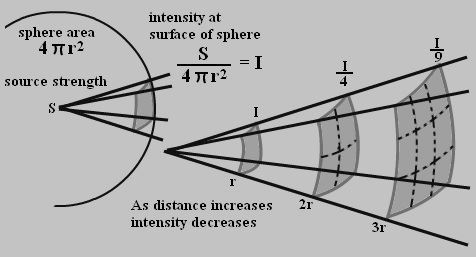# Inverse Square Law Formula

Inverse Square Law says that the strength of light (intensity) is proportional inversely to the square of the distance.## Formula of Inverse-Square Law

The inverse-square law formula is articulated as

$$I\propto \frac{1}{d^{2}}$$

Where,

• distance is d
• the intensity of the radiation is I.

At distances d1 and d2, I1 and I2 are intensities of light respectively. Then the Inverse-square law is articulated as:

$$\frac{I_{1}}{I_{2}}\propto \frac{d_{2}^{2}}{d_{1}^{2}}$$

The inverse-square law formula is handy in finding the distance or intensity of any given radiation. The intensity is articulated in Lumen or candela and distance is given in meters. It has widespread applications in problems grounded on the light.

### Inverse Square Law Solved Examples

Underneath are some problems based on an inverse square law which may be useful for you.

Problem 1: The intensity of monochromatic light is in the ratio 16:1. Calculate the second distance if the first distance is 6 m?

Known:

I1 : I2 = 16 : 1,

d1 = 6 m,

d2 =?

$$\frac{I_{1}}{I_{2}}\propto \frac{d_{2}^{2}}{d_{1}^{2}}$$

Distance, $$d_{2}=\sqrt{\frac{I_{1d_{1}^{2}}}{I_{2}}}$$

d2 =24 m
For more such valuable equations and formulas stay tuned with BYJU’S!!

#### 1 Comment

1. Q. If v1 and v2 are the linear velocities of a planet when it is respectively nearest and farthest from the sun, prove that
(1-e)v1=(1+e)v2.